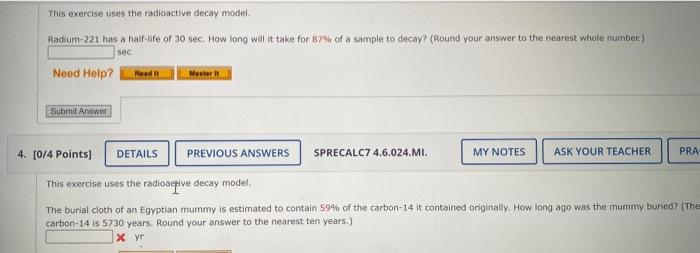# (Solved): This exercise uses the radioactive decay model. Radium-221 has a half-ife of 30 sec. How long will ...This exercise uses the radioactive decay model. Radium-221 has a half-ife of 30 sec. How long will it take for of a sample to decay? (Round your answer to the nearest whole number.) sec SPRECALC7 4.6.024.MI. This exercise uses the radioaceive decay model. The burial cloth of an Egyptian mummy is estimated to contain of the carbon-14 it contained originally. How long ago was the mummy buricd? (Th carbon-14 is 5730 years. Round your answer to the nearest ten years.) yr

We have an Answer from Expert